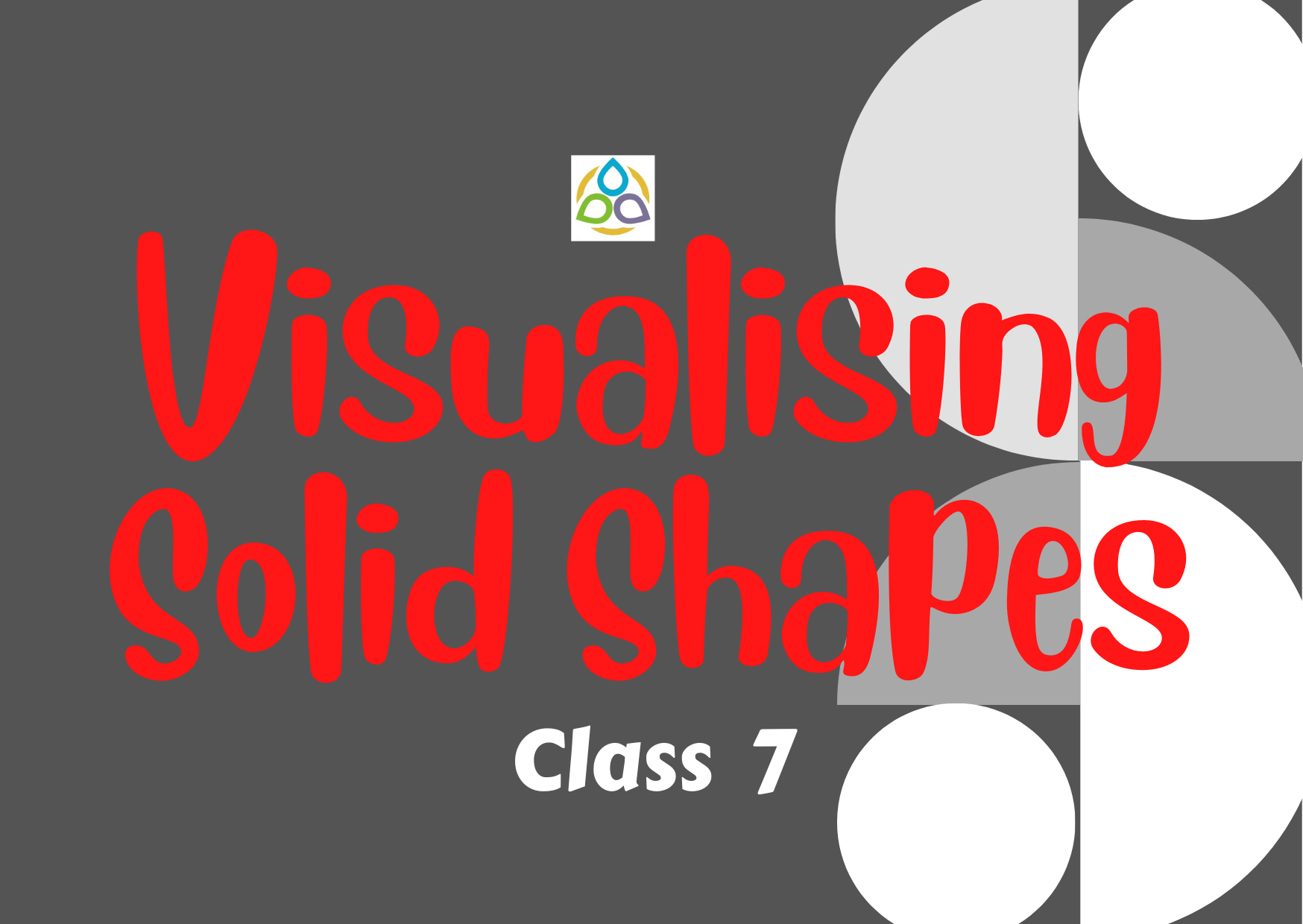# Visualising Solid Shapes – 7

15. In the following figure of a cube, how many edges that are parallel to the edge AB?

• (A) 4
• (B) 5
• (C) 2
• (D) 3

14. In the following figure of a cube, how many edges are perpendicular to the edge AB?

• (A) 4
• (B) 5
• (C) 2
• (D) 3

13. In the following figure of a cube, which three faces form the vertex H?

• (B) ABCD, HDCD, GHEA

12. Identical cubes are stacked in the corner of a room as shown below.

The number of cubes that are not visible are

• (A) 4
• (B) 5
• (C) 6
• (D) 7

11. Identical cubes are stacked in the corner of a room as shown below.

The number of cubes that are visible are

• (A) 4
• (B) 5
• (C) 6
• (D) 7

10. In the following figure, how many faces meeting at B?

• (A) 2
• (B) 3
• (C) 4
• (D) 5

9. The net given below can be used to make a cube. Which edge meets AN?

• (A) GH
• (B) IJ
• (C) KD
• (D) CD

8. The net given below can be used to make a cube. Which edge meets DE?

• (A) GF
• (B) IJ
• (C) LM
• (D) DC

7. If three cubes each of edge 4 cm are placed end to end, then the dimensions of resulting solid are:

• (A) 12 cm × 4 cm × 4 cm
• (B) 4 cm × 8 cm × 4 cm
• (C) 4 cm × 8 cm × 12 cm
• (D) 4 cm × 6 cm × 8 cm

6. If we rotate a right-angled triangle of height 5 cm and base 3 cm about its height a full turn, we get

• (A) cone of height 5 cm, base 3 cm
• (B) triangle of height 5 cm, base 3 cm
• (C) cone of height 5 cm, base 6 cm
• (D) triangle of height 5 cm, base 6 cm

5. If we rotate a right-angled triangle of height 5 cm and base 3 cm about its base, we get a

• (A) cone of height 3 cm and base 3 cm
• (B) cone of height 5 cm and base 5 cm
• (C) cone of height 5 cm and base 3 cm
• (D) cone of height 3 cm and base 5 cm

4. How many cubes are there in the following figure?

• (A) 21
• (B) 18
• (C) 19
• (D) None of these

3. How many cubes are there in the following figure?

• (A) 12
• (B) 14
• (C) 13
• (D) None of these

2. How many cubes are there in the following figure?

• (A) 16
• (B) 15
• (C) 12
• (D) None of these

1. How many cubes are there in the following figure?

• (A) 16
• (B) 15
• (C) 12
• (D) None of these